Next: Example 7.4: Energy in Up: Electric Current Previous: Example 7.2: Equivalent resistance

## Example 7.3: Kirchhoff's rules

Question: Find the three currents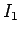,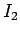, and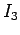in the circuit shown in the diagram, where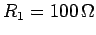,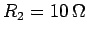,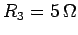,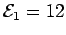V and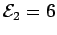V.

Answer: Applying the junction rule to point, and assuming that the currents flow in the direction shown (the initial choice of directions of the currents is arbitrary), we have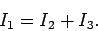There is no need to apply the junction rule again at point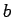, since if the above equation is satisfied then this rule is automatically satisfied at.Let us apply the loop rule by going around the various loops in the circuit in a clockwise direction. For loop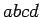, we have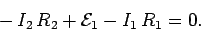Note that both the terms involving resistors are negative, since we cross the resistors in question in the direction of nominal current flow. Likewise, the term involving the emf is positive since we traverse the emf in question from the negative to the positive plate. For loop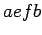, we find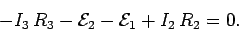There is no need to apply the loop rule to the full loop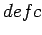, since this loop is made up of loopsand, and the loop rules for these two loops therefore already contain all of the information which would be obtained by applying the loop rule to.

Combining the junction rule with the first loop rule, we obtain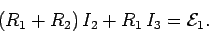The second loop rule can be rearranged to give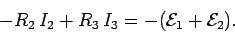The above two equations are a pair of simultaneous algebraic equations for the currentsand, and can be solved using the standard method for solving such equations. Multiplying the first equation by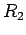, the second by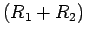, and adding the resulting equations, we obtain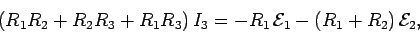which can be rearranged to give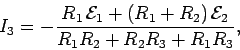or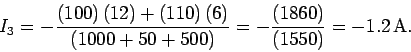Likewise, multiplying the first equation by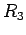, the second by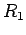, and taking the difference of the resulting equations, we obtain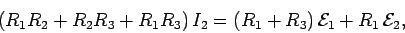which can be rearranged to give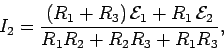or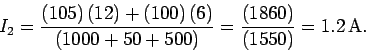Finally, from the junction rule,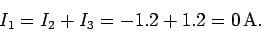The fact that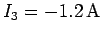indicates that this current is of magnitude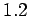A, but flows in the opposite direction to that which we initially guessed. In fact, we can see that a current ofA circulates in an anti-clockwise direction in the lower loop of the circuit, whereas zero current circulates in the upper loop.Next: Example 7.4: Energy in Up: Electric Current Previous: Example 7.2: Equivalent resistance
Richard Fitzpatrick 2007-07-14